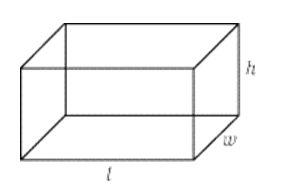# Rectangular Solids and Cylinders

 Rectangular SolidLearn the concepts behind volume and surface area before you start solving GMAT Solid geometry problems. All solid geometry problems come down to this - length, breadth and height. For data sufficiency questions, look out for values of l, b and h. if any of them are missing then it would be easy to eliminate answer choices.6 rectangular faces constitute a rectangular solid The formulas you need to remember for a rectangular solid areVolume = Length (l) x Width (w) x Height (h)Surface Area = (2 x Length x Width) + (2 x Length x Height) + (2 x Width x Height)"If length = width = height, that means that the rectangular solid is, in fact, a cube."TerminologiesVertex: Wow! quite a confusing word? Not reallyVertex = Cornera) Vertex is the number of corners in a...

Get F1GMAT's Newsletters (Best in the Industry)

• Ranking Analysis
• Post-MBA Salary Trends
• Post-MBA Job Function & Industry Analysis
• Post-MBA City Review
• MBA Application Essay Tips
• School Specific Essay Tips
• GMAT Preparation Tips